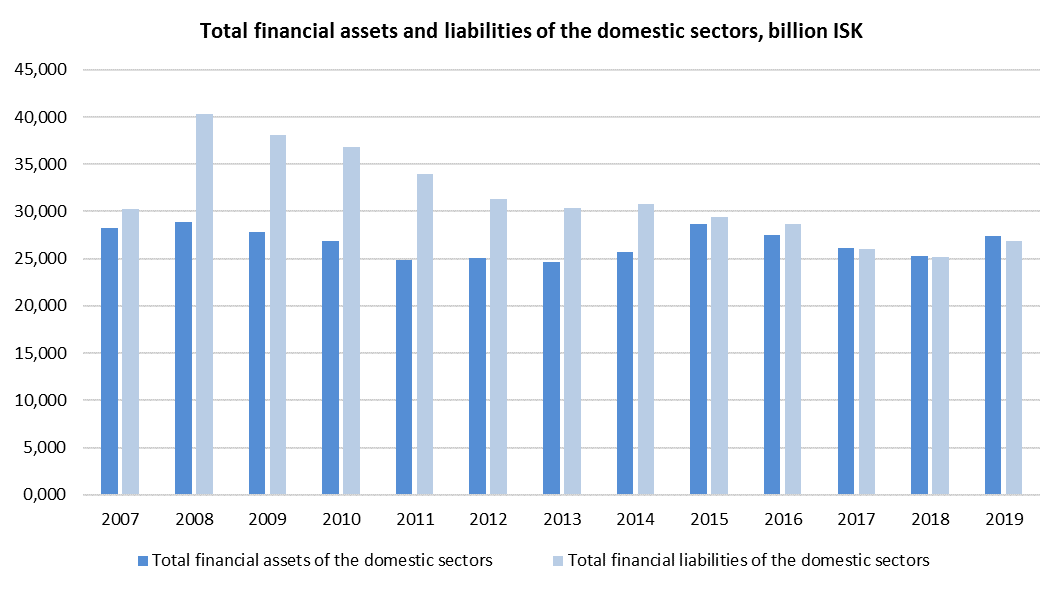Total financial assets of Iceland’s domestic sector stood at 27,444 billion ISK or 901% of Gross Domestic Product (GDP) in year-end 2019. Total financial liabilities stood at 26,925 billion ISK or 884% of GDP according to newly updated figures from Statistics Iceland.Financial assets of households stood at 7,962 billion ISK, while financial liabilities stood at 2,294 billion ISK at the end of 2019, or 261% and 75% of GDP, respectively.

Financial assets of non-financial corporations stood at 3,145 billion ISK according to the revised data, while liabilities stood at 6,828 billion ISK.

Total financial assets of financial corporations stood at 13,829 billion ISK, while liabilities stood at 14,672 billion ISK at the end of 2019.

At the end of 2019, the financial assets of the general government stood at 2,410 billion ISK, or 79% of GDP, while liabilities stood at 3,122 billion ISK, or 103% of GDP.

The foreign sector’s financial assets stood at 3,625 billion ISK, or 119% of GDP, while the liabilities stood at 4,132 billion ISK, or 136% of GDP.

The next publication of financial accounts will be in September 2021.

Statistics